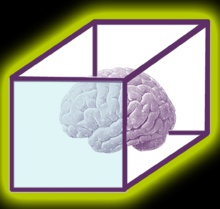'Recognizing the need is the primary condition for design.'

Charles Eames

'To design is to communicate clearly by whatever means you can control or master.'
Milton Glaser

# Unit 6 Electrical and Electronic Principles

Unit 6 Old Spec 2010 ELectrical and Elec[...]

On completion of this unit a learner should:

1 Be able to use circuit theory to determine voltage, current and resistance in direct current (DC) circuits

2 Understand the concepts of capacitance and determine capacitance values in DC circuits

3 Know the principles and properties of magnetism

4 Be able to use single-phase alternating current (AC) theory.

# P1 & P2 Use DC circuit theory to calculate current, voltage and resistance in a DC network.

Fig 1: Battery powering two light bulbs of unequal resistance.

When current is flowing through the light bulb, the resistance of the filament causes a loss of voltage that is proportional to the resistance and the amount of current. We refer to this as the voltage across the bulb or as the bulb’s voltage drop.

Fig 2: Voltmeters are used to measure the voltage across the light bulbs

We see that the voltage across light bulb A is 2V and the voltage across light bulb B is 1V.

Next, we’ll measure the current.

Fig 3: Figure 5. Inset an ammeter such that the current flowing through the light bulbs flows into one probe, through the device’s current-measuring circuitry, and out the other probe.

Let’s assume that we measure 1A. We have now made the measurements that we need in order to determine the power dissipation of the light bulbs.

How to calculate the power dissipated by each light bulb.

Insert the measured values into the formula given above.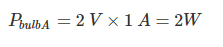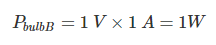If we want to know the power dissipated by the entire circuit, we add up the power dissipation of the individual components: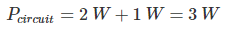Or, we can multiply the current supplied from the battery by the battery’s voltage: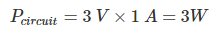Below is an introduction to Ohm’s law, which expresses the fundamental relationship between current, voltage, and resistance.

June 2020

# P3 Compere the forward and reverse charachteristics of two different types of semi conductor diode.

A diode is two terminal electronics component, which can be connected to the source in two different ways; Forward Bias and Reverse Bias. The forward bias works like a closed switch and allows the current through itself. In contrast to forward bias, the reverse bias connection blocks the current passage and works as an open switch. The biasing of a diode depends upon the direction of the source. Ref: https://electric-shocks.com/forward-and-reverse-bias-of-diode-explained-by-v-i-characteristic-curves/

Below are three diagrams that describe forward and reverse charachteristics of diodes.

# P4 Types and functions of capacitors

Polar and Non-Polar Capacitor:

Non Polar Capacitor: (Used in both AC and DC Systems)
The Non Polar capacitors can be used in both AC and DC systems. They can be connected to the power supply in any direction and their capacitance does not effect by the reversal of polarity.

Polar Capacitor: (Only used in DC Circuits and Systems)
This type of capacitor is sensitive about their polarity and can be only used in DC systems and networks. Polar Capacitors don’t work in the AC system, because of the reversal of polarity after each half cycle in AC supply.

Capacitor applications in AC systems:

Transformer less power supply:
Capacitors are used in transformer less power supplies. In such circuits, the capacitor is connected in series with the load because we know that the capacitor and inductor in pure form does not consume power. They just take power in one cycle and deliver it back in the other cycle to the load. In this case, it is used to reduce the voltage with less power wastage.

Split phase induction motors:
The capacitors are also used in induction motor to split a single phase supply into a two phase supply to produce a revolving magnetic field in the rotor to catch that field. This type of capacitor is mostly used in household water pumps, Fans, air conditioner and many devices which need at least two phases to work.

Power Factor Correction and Improvement:
There are lots of advantages of power factor improvement. In a three phase power systems, capacitor bank is used to supply reactive power to the load and hence improve the power factor of the system. Capacitor bank is installed after a precise calculation. Basically, it delivers the reactive power which was previously traveled from the power system, hence it reduces the losses and improves the efficiency of the system.

Capacitors in DC Circuits and systems:

Power conditioning:
In DC systems, capacitor is used as a filter (mostly). Its most common use is converting AC to DC power supply in rectification (such as bridge rectifier). When AC power is converted into fluctuating (with ripples i.e. not a steady state with the help of rectifier circuits) DC power (Pulsating DC) in order to smooth and filter out these ripples and fluctuation, DC Polar capacitor is used. Its value is calculated precisely and depends on the system voltage and the demand load current.

Decoupling Capacitor:
Decoupling capacitor is used, where we have to decouple the two electronics circuits. In other words, the noise generated by one circuit is grounded by decoupling capacitor and it does not affect the performance of other circuit.

Coupling Capacitor:
As we know that Capacitor blocks DC and allows AC to flow through it (we will discuss it in the next session that how does it happens). So it is used to separate AC and DC signals (also used in the filter circuits for the same purpose). Its value is calculated in such a way that its reactance is minimized on the basis of frequency, which we want to pass through it. Coupling Capacitor is also used in filters (ripple remover circuits like RC filters) to separate AC and DC signal and removes the ripples from pulsating DC supply voltage to convert it into pure AC voltage after rectification.

# P8 Describe the relationship between flux density and field strength

As previously mentioned, permeability (m) is a material property that describes the ease with which a magnetic flux is established in a component. It is the ratio of the flux density (B) created within a material to the magnetizing field (H) and is represented by the following equation: m = B/H

The shape of the hysteresis loop tells a great deal about the material being magnetized. The hysteresis curves of two different materials are shown in the graph.

Relative to other materials, a material with a wider hysteresis loop has:

• Lower Permeability
• Higher Retentivity
• Higher Coercivity
• Higher Reluctance
• Higher Residual Magnetism

Relative to other materials, a material with the narrower hysteresis loop has:

• Higher Permeability
• Lower Retentivity
• Lower Coercivity
• Lower Reluctance
• Lower Residual Magnetism.

In magnetic particle testing, the level of residual magnetism is important. Residual magnetic fields are affected by the permeability, which can be related to the carbon content and alloying of the material. A component with high carbon content will have low permeability and will retain more magnetic flux than a material with low carbon content.

In the two B-H loops above, which one would indicative of a low carbon steel??

# M1 - use Kirchhoff’s laws to determine the current in various parts of a network having four nodes and the power dissipated in a load resistor containing two voltage sources

## What is a load resistor in circuit?

Below are those things that can be load resistance:

1) Simple resistor
2) Audio speakers
3) LED lights
4) All types of the light indicator
5) Resistive sensors
6) Buzzers
7) The output stage of the radio frequency amplifier or audio frequency amplifier.
8) Antennas
9) High wattage resistor

Kirchhoff's Current Law
The total of the current that flows into a junction point of a circuit is equal to the total amount of current that flows out of that same junction.

Kirchhoff's Voltage Law
The electric potential difference (voltage) of the battery (energy source) is equal to the total of the electric potential differences of the loads combined.

Example of a 4 node circuit with 2 voltage sources you could use to design and simulate a circuit for M1

# M2 - evaluate capacitance, charge, voltage and energy in a network containing a seriesparallel combination of three capacitors

Series, Parallel and Series / Parallel combination circuit to use in your simulations and calculations for M2

Useful calculators for charge. capatacence, voltage and energy.